# Texas Go Math Grade 5 Lesson 6.1 Answer Key Find Part of a Group

Refer to our Texas Go Math Grade 5 Answer Key Pdf to score good marks in the exams. Test yourself by practicing the problems from Texas Go Math Grade 5 Lesson 6.1 Answer Key Find Part of a Group.

## Texas Go Math Grade 5 Lesson 6.1 Answer Key Find Part of a Group

Unlock the Problem

Maya collects stamps. She has 20 stamps in her collection. Four-fifths of her stamps have been canceled. How many of the stamps in Maya’s collection have been canceled?

Find $$\frac{4}{5}$$ of 20.

• Put 20 counters on your Math Board.
Since you want to find $$\frac{4}{5}$$ of the stamps, you should arrange the 20 counters in ________ equal groups.
• Draw the counters in equal groups below. How many counters are in each group? _________Each group represents __________ of the stamps. Circle $$\frac{4}{5}$$ of the counters.
How many groups did you circle? ___________
How many counters did you circle? ___________
$$\frac{4}{5}$$ of 20 = __________ , or $$\frac{4}{5}$$ × 20 = ______
So, _________ of the stamps have been canceled.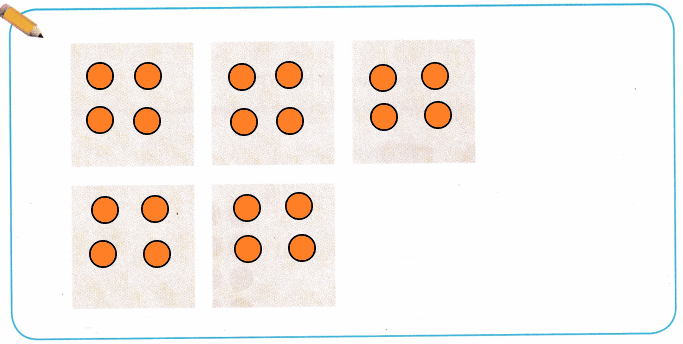Put 20 counters on above Math Board.
Since I want to find 4/5 of the stamps, I should arrange the 20 counters in 5 equal groups.
Draw the counters in equal groups above. There are 4 counters are in each group.
Each group represents 1/5 of the stamps. Draw a circle 4/5 of the counters.
I circled 4 groups.
I circled 16 counters.
4/5 of 20 =16, or 4/5 × 20 = 16.
So, 16 of the stamps have been canceled.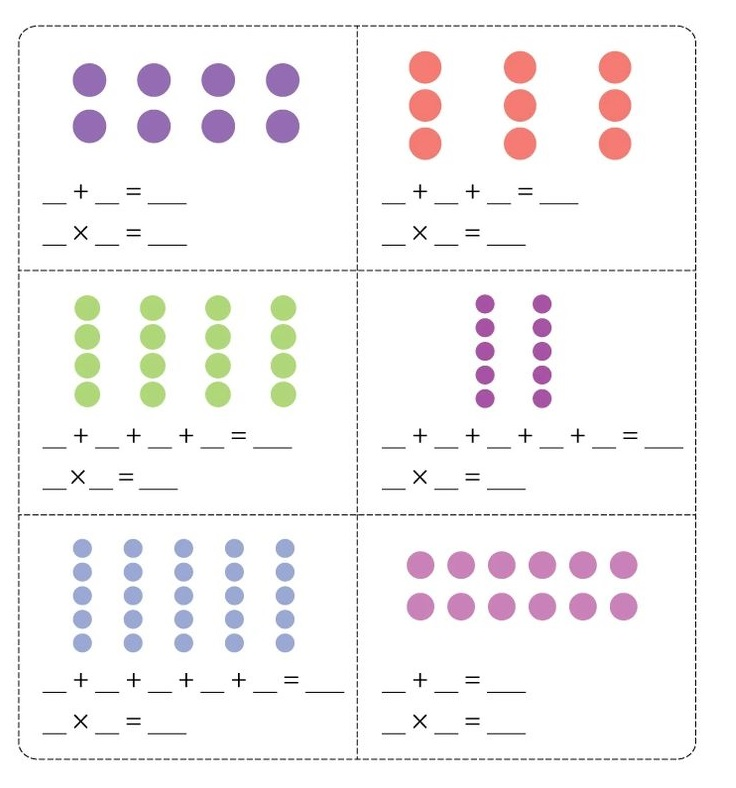Example

Max’s stamp collection has stamps from different countries. He has 12 stamps from Canada. Of those twelve, $$\frac{2}{3}$$ of them have pictures of Queen Elizabeth II. How many stamps have the queen on them?

Draw an array to represent the 12 stamps by drawing an ✗ for each stamp. Since you want to find $$\frac{2}{3}$$ of the stamps, your array should show __________ rows of equal size.

Circle _________ of the 3 rows to show $$\frac{2}{3}$$ of 12. Then count the number of ✗s in the circle.
There are _________ ✗s circled.

Complete the number sentences.
$$\frac{2}{3}$$ of 12 = ______, or $$\frac{2}{3}$$ × 12 = _________
So, there are __________ stamps with a picture of Queen Elizabeth II.
Draw an array to represent the 12 stamps by drawing an ✗ for each stamp. Since we want to find 2/3 of the stamps, our array should show 3 rows of equal size.Circle 2 of the 3 rows to show 2/3 of 12. Then count the number of ✗s in the circle.
There are 8 ✗s circled.
The number sentences.
2/3 of 12 = 8, or 2/3× 12 = 8
So, there are 8 stamps with a picture of Queen Elizabeth II.

Share and Show

Question 1.
Complete the model to solve.
$$\frac{7}{8}$$ of 16, or $$\frac{7}{8}$$ × 16a. How many rows of counters are there?
There are 8 rows of counters.

b. How many counters are in each row?
There are 2 counters are in each row.

c. Circle rows to solve the problem.Circle 7 rows to solve the problem.

d. How many counters are circled?
$$\frac{7}{8}$$ of 16 = _________, or $$\frac{7}{8}$$ × 16 = __________
14 counters are circled.
7/8 of 16 = 14 or (7/8) x 16 = 14

Use a model to solve.

Question 2.
$$\frac{2}{3}$$ × 18 = __________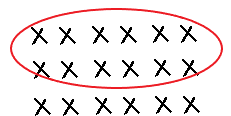(2/3) x 18 = 12
Explanation:
In the above image, we can observe an array to represent the 18 counters by drawing an ✗ for each counter. Since we want to find 2/3 of the counters, our array should show 3 rows of equal size. Circle 2 of the 3 rows to show 2/3 x 18. Then count the number of ✗s in the circle. There are 12 ✗s circled. The number sentence is (2/3) x 18 = 12.

$$\frac{2}{5}$$ × 15 = __________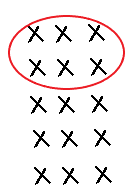(2/5) x 15 = 6
Explanation:
In the above image we can observe an array to represent the 15 counters by drawing an ✗ for each counter. Since we want to find 2/5 of the counters, our array should show 5 rows of equal size. Circle 2 of the 5 rows to show 2/5 x 15. Then count the number of ✗s in the circle. There are 6 ✗s circled. The number sentence is (2/5) x 15 = 6.

Question 4.
$$\frac{2}{3}$$ × 6 = __________(2/3) x 6 = 4
Explanation:
In the above image we can observe an array to represent the 6 counters by drawing an ✗ for each counter. Since we want to find 2/3 of the counters, our array should show 3 rows of equal size. Circle 2 of the 3 rows to show 2/3 x 6. Then count the number of ✗s in the circle. There are 4 ✗s circled. The number sentence is (2/3) x 6 = 4.

Problem Solving

Use a model to solve.

Question 5.
$$\frac{5}{8}$$ × 24 = __________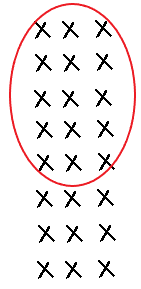(5/8) x 24 = 15
Explanation:
In the above image we can observe an array to represent the 24 counters by drawing an ✗ for each counter. Since we want to find 5/8 of the counters, our array should show 8 rows of equal size. Circle 5 of the 8 rows to show
5/8 x 24. Then count the number of ✗s in the circle. There are 15 ✗s circled. The number sentence is (5/8) x 24 = 15.

Question 6.
$$\frac{3}{4}$$ × 24 = __________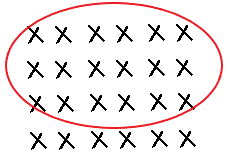(3/4) x 24 = 18
Explanation:
In the above image we can observe an array to represent the 24 counters by drawing an ✗ for each counter. Since we want to find 3/4 of the counters, our array should show 4 rows of equal size. Circle 3 of the 4 rows to show
3/4 x 24. Then count the number of ✗s in the circle. There are 18 ✗s circled. The number sentence is (3/4) x 24 = 18.

$$\frac{4}{7}$$ × 21 = __________(4/7) x 21 = 12
Explanation:
In the above image we can observe an array to represent the 21 counters by drawing an ✗ for each counter. Since we want to find 4/7 of the counters, our array should show 7 rows of equal size. Circle 4 of the 7 rows to show
4/7 x 21. Then count the number of ✗s in the circle. There are 12 ✗s circled. The number sentence is (4/7) x 21 = 12.

Question 8.
On your Math Board, use counters to find $$\frac{4}{6}$$ of 12. Explain why the answer is the same as when you found $$\frac{2}{3}$$ of 12.They are the same because (4/6) = (2/3).
Explanation:
On the above Math Board 12 counters in 6 rows. In each row there are 2 counters. Draw a circle for 8 counters. The number sentences for (4/6) of 12 is 8 and (2/3) of 12 is 8. So both are because (4/6) is equal to (2/3).

Problem Solving

Use the table for 9 – 10.Question 9.
Representations Four-fifths of Zack’s stamps have pictures of animals. How many stamps with pictures of animals does Zack have? Use a model to solve.(4/5) of 30 = 24
Zack has 24 stamps with pictures of animals.
Explanation:
In the above table, we can observe that Zack collected 30 stamps. Draw an array to represent the 30 stamps by drawing an ✗ for each stamp. Four-fifths of Zack’s stamps have pictures of animals. Since we want to find 4/5 of the stamps, our array should show 5 rows of equal size. Circle 4 of the 5 rows to show 4/5 x 30. Then count the number of ✗s in the circle. There are 24 ✗s circled. The number sentence is (4/5) of 30 = 24. Zack have 24 stamps with pictures of animals.

H.O.T. Write Math Zack, Teri, and Paco combined the foreign stamps from their collections for a stamp show. Out of their collections, $$\frac{3}{10}$$ of Zack’s stamps, $$\frac{5}{6}$$ of Teri’s stamps, and $$\frac{3}{8}$$ of Paco’s stamps were from foreign countries. How many stamps were in their display? Explain how you solved the problem.9 + 15 + 9 = 33
In the display there are 33 stamps.
Explanation:
Zack, Teri, and Paco combined the foreign stamps from their collections for a stamp show. Out of their collections, 3/10 of Zack’s stamps, 5/6 of Teri’s stamps, and 3/8 of Paco’s stamps were from foreign countries. Multiply 3/10 with 30 the product is 9. Zack’s stamps are 9. Multiply 5/6 with 18 the product is 15. Teri’s stamps are 15. Multiply 3/8 with 24 the product is 9. Paco’s stamps are 9. I found the fractional part of each persons collection. I added the amounts together. So, 9 + 15 + 9 = 33.

Question 11.
Multi-Step Paula has 24 stamps in her collection. Among her stamps, $$\frac{1}{3}$$ have pictures of animals. Out of her stamps with pictures of animals, $$\frac{3}{4}$$ of those stamps have pictures of birds. How many stamps have pictures of birds on them?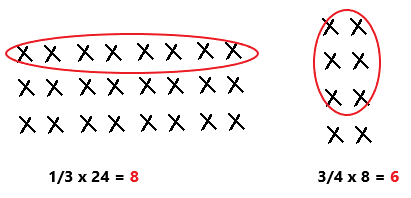6 stamps have pictures of birds on them.
Explanation:
Paula has 24 stamps in her collection. Among her stamps, 1/3 have pictures of animals. Multiply 1/3 with 24 the product is 8. 8 stamps have pictures of animals. Out of her stamps with pictures of animals,3/4 of those stamps have pictures of birds. Multiply 3/4 with 8 the product is 6. 6 stamps have pictures of birds on them.

Question 12.
Apply Eduardo has 30 pairs of socks in a drawer. Of those, 6 pairs are matched and the rest are mismatched. He is packing 1/2 of his mismatched pairs in his suitcase for a trip. How many pairs is Eduardo packing for his trip?
(A) 6
(B) 4
(C) 12
(D) 1830 – 6 = 24
There are 24 mismatched pairs of socks in a drawer.
1/2 x 24 = 12
Eduardo packing 12 mismatched pairs of socks for his trip.
So, option C is correct.
Explanation:
Eduardo has 30 pairs of socks in a drawer. Of those, 6 pairs are matched and the rest are mismatched. Subtract 6 from 30 the difference is 24. There are 24 mismatched pairs of socks in a drawer. He is packing 1/2 of his mismatched pairs in his suitcase for a trip. Multiply 1/2 with 24 the product is 12. Eduardo packing 12 mismatched pairs of socks for his trip. So, draw a circle to option C.

Use Diagrams Which problem does the model represent?(2/3) x 15 = 10
So, option A is correct.
Explanation:
In the above we can observe 15 counters. Drawn a rectangle to 2 columns. So, the problem (2/3) x 15 represents the above model diagram. So, draw a circle for option A.

Question 14.
Multi-Step Amy has 25 different hair ribbons. She bought 4 of them in a store, but she made the rest herself. Of the hair ribbons she made, $$\frac{1}{3}$$ have sparkles on them. How many hair ribbons with sparkles did Amy make?
(A) 9
(B) 7
(C) 3
(D) 14

Texas Test Prep

Question 15.
Barry bought 21 stamps from a hobby shop. He gave $$\frac{3}{7}$$ of them to his sister. How many stamps did he have left?
(A) 9 stamps
(B) 6 stamps
(C) 3 stamps
(D) 12 stamps(3/7) x 21 = 9
He gave 9 stamps to his sister.
21 – 9 = 12
Barry left with 12 stamps.
So, option A is correct.
Explanation:
Barry bought 21 stamps from a hobby shop. He gave 3/7 of them to his sister. Multiply 3/7 with 21 the product is 9. He gave 9 stamps to his sister. Subtract 9 from 21 the difference is 12. Barry left with 12 stamps. So, draw a circle to option A.

### Texas Go Math Grade 5 Lesson 6.1 Homework and Practice Answer Key

Use a model to solve.

Question 1.
$$\frac{3}{5}$$ × 10 = __________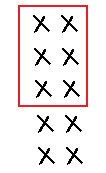(3/5) x 10 = 6
Explanation:
In the above image we can observe an array to represent the 10 counters by drawing an ✗ for each counter. Since we want to find 3/5 of the counters, our array should show 5 rows of equal size. Circle 3 of the 5 rows to show
3/5 x 10. Then count the number of ✗s in the circle. There are 6 ✗s circled. The number sentence is (3/5) x 10 = 6.

$$\frac{2}{3}$$ × 15 = __________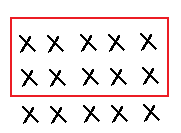(2/3) x 15 = 10
Explanation:
In the above image we can observe an array to represent the 15 counters by drawing an ✗ for each counter. Since we want to find 2/3 of the counters, our array should show 3 rows of equal size. Circle 2 of the 3 rows to show
2/3 x 15. Then count the number of ✗s in the circle. There are 10 ✗s circled. The number sentence is (2/3) x 15 = 10.

Question 3.
$$\frac{3}{8}$$ × 16 = __________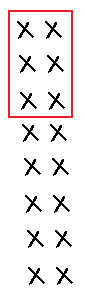(3/8) x 16 = 6
Explanation:
In the above image we can observe an array to represent the16 counters by drawing an ✗ for each counter. Since we want to find 3/8 of the counters, our array should show 8 rows of equal size. Circle 3 of the 8 rows to show
3/8 x 16. Then count the number of ✗s in the circle. There are 6 ✗s circled. The number sentence is (3/8) x 16 = 6.

Question 4.
$$\frac{5}{6}$$ × 30 = __________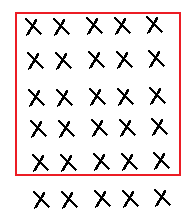(5/6) x 30 = 25
Explanation:
In the above image we can observe an array to represent the 30 counters by drawing an ✗ for each counter. Since we want to find 5/6 of the counters, our array should show 6 rows of equal size. Circle 5 of the 6 rows to show
5/6 x 30. Then count the number of ✗s in the circle. There are 25 ✗s circled. The number sentence is (5/6) x 30 = 25.

Question 5.
$$\frac{5}{7}$$ × 14 = __________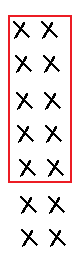(5/7) x 14 = 10
Explanation:
In the above image we can observe an array to represent the 14 counters by drawing an ✗ for each counter. Since we want to find 5/7 of the counters, our array should show 7 rows of equal size. Circle 5 of the 7 rows to show
5/7 x 14. Then count the number of ✗s in the circle. There are 10 ✗s circled. The number sentence is (5/7) x 14 = 10.

Question 6.
$$\frac{3}{5}$$ × 25 = __________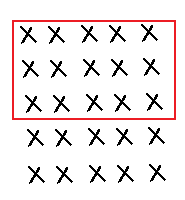(3/5) x 25 = 15
Explanation:
In the above image we can observe an array to represent the 25 counters by drawing an ✗ for each counter. Since we want to find 3/5 of the counters, our array should show 5 rows of equal size. Circle 3 of the 5 rows to show
3/5 x 25. Then count the number of ✗s in the circle. There are 15 ✗s circled. The number sentence is (3/5) x 25 = 15.

Question 7.
$$\frac{3}{4}$$ × 16 = __________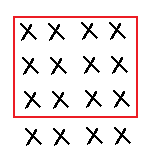(3/4) x 16 = 12
Explanation:
In the above image we can observe an array to represent the 16 counters by drawing an ✗ for each counter. Since we want to find 3/4 of the counters, our array should show 4 rows of equal size. Circle 3 of the 4 rows to show
3/4 x 16. Then count the number of ✗s in the circle. There are 12 ✗s circled. The number sentence is (3/4) x 16 = 12.

Question 8.
$$\frac{2}{5}$$ × 20 = __________(2/5) x 20 = 8
Explanation:
In the above image, we can observe an array to represent the 20 counters by drawing an ✗ for each counter. Since we want to find 2/5 of the counters, our array should show 4 rows of equal size. Circle 2 of the 5 rows to show
2/5 x 20. Then count the number of ✗s in the circle. There are 8 ✗s circled. The number sentence is (2/5) x 20 = 8.

$$\frac{4}{7}$$ × 35 = __________(4/7) x 35 = 20
Explanation:
In the above image we can observe an array to represent the 35 counters by drawing an ✗ for each counter. Since we want to find 4/7 of the counters, our array should show 7 rows of equal size. Circle 4 of the 7 rows to show
4/7 x 35. Then count the number of ✗s in the circle. There are 20 ✗s circled. The number sentence is (4/7) x 35 = 20.

Question 10.
$$\frac{2}{3}$$ × 21 = __________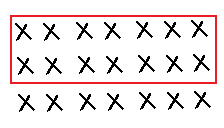(2/3) x 21 = 14
Explanation:
In the above image we can observe an array to represent the 21 counters by drawing an ✗ for each counter. Since we want to find 2/3 of the counters, our array should show 3 rows of equal size. Circle 2 of the 3 rows to show
2/3 x 21. Then count the number of ✗s in the circle. There are 14 ✗s circled. The number sentence is (2/3) x 21 = 14.

Question 11.
$$\frac{3}{4}$$ × 28 = __________(3/4) x 28 = 21
Explanation:
In the above image we can observe an array to represent the 28 counters by drawing an ✗ for each counter. Since we want to find 3/4 of the counters, our array should show 4 rows of equal size. Circle 3 of the 4 rows to show
3/4 x 28. Then count the number of ✗s in the circle. There are 21 ✗s circled. The number sentence is (3/4) x 28 = 21.

Question 12.
$$\frac{8}{9}$$ × 27 = __________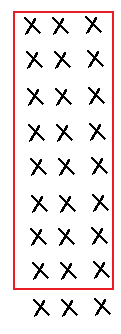(8/9) x 27 = 24
Explanation:
In the above image we can observe an array to represent the 27 counters by drawing an ✗ for each counter. Since we want to find 8/9 of the counters, our array should show 9 rows of equal size. Circle 8 of the 9 rows to show
8/9 x 27. Then count the number of ✗s in the circle. There are 24 ✗s circled. The number sentence is (8/9) x 27 = 24.

Question 13.
Will the product of a fraction less than one and a whole number be less than or greater than the whole number? Explain.
It can be either.
1/2 * 4 = 2, so the answer is less.
-1/2 * 4 = -2, so the answer is less.
1/2 * -4 = -2, so the answer is greater.
-1/2 * -4 = 2, so the answer is greater.

Problem Solving

Question 14.
Lauren spent 24 hours on the computer last week. She spent $$\frac{2}{3}$$ of the time doing homework. How much time did Lauren spend doing homework?
(2/3) x 24 = 16
Lauren spend 16 hours doing homework.
Explanation:
Lauren spent 24 hours on the computer last week. She spent (2/3) of the time doing homework. Multiply (2/3) with 24 the result is 16. Lauren spend 16 hours doing homework.

Question 15.
A display at the natural science museum contains 21 plant and animal fossils. $$\frac{4}{7}$$ of the fossils in the display are animal fossils. How many fossils in the display are animal fossils?
(4/7) x 21 = 12
12 fossils in the display are animal fossils.
Explanation:
A display at the natural science museum contains 21 plant and animal fossils. (4/7) of the fossils in the display are animal fossils. Multiply 4/7 with 21 the product is 12. So,12 fossils in the display are animal fossils.

Lesson Check

Question 16.
Today, the fifth-grade class will explore all 36 fossil displays at the museum. They explore $$\frac{4}{9}$$ of the displays in the morning. How many displays are left for the class to explore in the afternoon?
(A) 20
(B) 16
(C) 18
(D) 32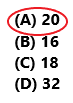(4/9) x 36 = 16
36 – 16 = 20
20 displays are left for the class to explore in the afternoon.
So, option A is correct.
Explanation:
Today, the fifth-grade class will explore all 36 fossil displays at the museum. They explore(4/9) of the displays in the morning. Multiply 4/9 with 36 the product is 16. They explore 16 displays in the morning. Subtract 16 from 36 the difference is 20. So, 20 displays are left for the class to explore in the afternoon. Draw a circle to option A.

Question 17.
There are 32 students in Mr. Samuelson’s class. $$\frac{5}{8}$$ of the students are boys. How many of the students in the class are girls?
(A) 27
(B) 3
(C) 20
(D) 12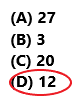(5/8) x 32 = 20
32 – 20 = 12
There are 12 students in the class are girls.
So, option D is correct.
Explanation:
There are 32 students in Mr. Samuelson’s class. 5/8 of the students are boys. Multiply 5/8 with 32 the product is 20. So, 20 students in the class are boys. Subtract 20 from 32 the difference is 12. There are 12 students in the class are girls. So draw a circle for option D.

Miguel uses counters to solve $$\frac{5}{8}$$ × 16.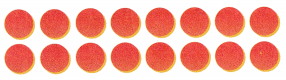How many counters will Miguel circle for the product?
(A) 10
(B) 6
(C) 5
(D) 12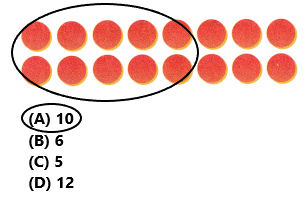(5/8) x 16 = 10
Miguel will circle 10 counters for the product.
So, option A is correct.
Explanation:
Miguel uses above counters to solve (5/8) × 16. In the above image we can observe 2 rows with 16 counters. Multiply 5/8 with 16 the product is 10. Miguel will circle 10 counters for the product. So, option A is correct.

Question 19.
Which problem does the model represent?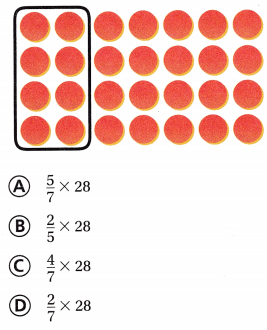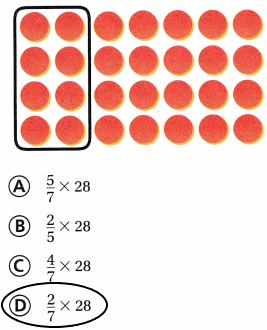(2/7) x 28 = 8
So, option D is correct.
Explanation:
In the image we can observe 28 counters with 4 rows. Multiply 2/7 with 28 the product is 8. So, option D (2/7) x 28 represents the above problem.

Question 20.
Multi-Step The members of the parents’ association are making 6 batches of brownies and 12 batches of fruit bars for the bake sale. They need $$\frac{1}{2}$$ cup of sugar for each batch. How much sugar do they need?
(A) 9 cups
(B) 36 cups
(C) 3 cups
(D) 6 cups1/2 x (6 + 12)
1/2 x 18
9
They need 9 cups of sugar.
So, option A is correct.
Explanation:
The members of the parents’ association are making 6 batches of brownies and 12 batches of fruit bars for the bake sale. They need 1/2 cup of sugar for each batch. Multiply 1/2 with 6 and 12 the product is 9. They need 9 cups of sugar. So, draw a circle to option A.

Question 21.
Multi-Step Natalie had 64 beads. She used 24 beads to make a bracelet. She used $$\frac{7}{8}$$ of the remaining beads to make a necklace. How many beads does Natalie have left?
(A) 40
(B) 21
(C) 5
(D) 35